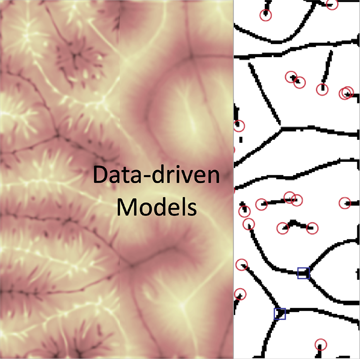## Thermal convectionConvection processes can be found almost everywhere. Think of air condition systems in buildings, the generation of magnetic fields in the Earth and Sun, or the formation of clouds in the atmosphere. The figure is a view from the top onto a cylindrical Rayleigh-Benard convection cell, one of the simplest convection systems. The streamlines of the instantaneous velocity field are plotted. They indicate a complex large-scale circulation pattern which evolves in convective turbulence. One of the big miracles in convection is how these patterns grow out of the stochastic turbulent fluid motion and how they affect the turbulent heat transport through the cell. A way to attempt this problem is by a drastic reduction of the degrees of freedom in the underlying mathematical model. Such a so-called low-dimensional system can then be analysed with very powerful instruments of the theory of dynamical systems.

Furthermore, we study the impact of moisture on thermal convection which allows for additional condensation and evaporation. This aspect is important for a better understanding of the formation, shape and lifetime of atmospheric clouds.

Current Collaborations:
Janet D. Scheel (Occidental College Los Angeles),
Holger Siebert (Leibniz-Institut für Troposphärenforschung Leipzig),
Raymond A. Shaw (Michigan Technological University Houghton),
Olivier Pauluis (Courant Institute for Mathematical Sciences, New York University)

## MagnetohydrodynamicsIn metallurgical applications, magnetic fields can be used to  brake, stir and control the flow of liquid metals. Examples include the continuous casting of steel or crystal growth, where both static and alternating fields are routinely applied. An understanding of the physics of such magnetohydrodynamic flows can be obtained by accurate numerical simulations. We are interested in the transition to turbulence in simple wall-bounded flows in channels, ducts and pipes, which we study by stability analyses and direct numerical simulations. Simulations are also used to investigate properties of developed turbulence in such flows. For most cases, the magnetic field has no independent dynamics because magnetic diffusion is dominant (quasistatic limit).

In the near future we shall also employ the full induction equation for flows with rapid transients. For the simulations we use our own spectral- and finite-difference  methods on structured meshes that allow for an efficient parallelization.

Current Collaborations:
Oleg Zikanov (University of Michigan Dearborn)
Leo Bühler (Karlsruher Institut für Technologie)

## Data-driven models and machine learningComplex dynamical systems such as turbulent flows are characterized by a huge number of degrees of freedom, i.e., independent variables that describe the temporal evolution. Strictly speaking, this number is infinitely large. In many application cases the number of degrees of freedom has to be reduced significantly to build smaller dynamical model that describe the time evolution of the most dominant degrees of freedom and to model the turbulent flow effectively. Turbulent flows are a prominent example. This is done in several ways such as by machine learning algorithms, Markov state models or Koopman operator analysis in the Eulerian frame of reference or by spectral and evolutionary clustering methods of fluid parcel trajectories in the Lagrangian frame of reference of fluid description.

Current Collaborations:
Peter Koltai (Freie Universität Berlin)
Dimitris Giannakis (New York University)
Carl Zeiss Foundation project DeepTurb (TU Ilmenau)

## Fluid TurbulenceThe figure shows an isovolume plot of the vorticity magnitude in a direct numerical simulation of homogeneous isotropic turbulence on a computational grid of about 10 billion grid points. Visible are elongated structures, so-called vortex tubes. One fundamental question is related to the growth rates of such vortex filaments. In addition, we study the mixing of active and passive scalar fields in turbulence and the decay of large-scale anisotropies in a turbulent flow. The latter is important for the development of subgrid-scale models of turbulence that are frequently necessary in computational fluid dynamics.

Current Collaborations:
Katepalli R. Sreenivasan (New York University),
Victor Yakhot (Boston University)

## Quantum computingQuantum computing changes simulation methods and data analyses in several ways as the algorithms follow the fundamental laws of quantum mechanics. Quantum bits or in short qubits are the smallest information unit on a quantum computer similar to bits on a classical one. We are interested in the modelling of (nonlinear) classical dynamical systems such as fluid flows on quantum computers as the number of degrees of freedom that can be explored in a simulation on a quantum computer can grow exponentially with the number of qubits. This property also opens new roads for machine learning algorithms which we explore in our group.

Current collaborations:

Joanna Slawinska (University of Helsinki)
Dimitris Giannakis (New York University)
Abbas Ourmazd (University of Wisconsin-Milwaukee)